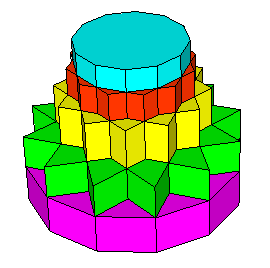A quiz to see how much you know about shapes and angles. Lets see how you do!

What are the interior angles (in degrees) of a regular hexagon?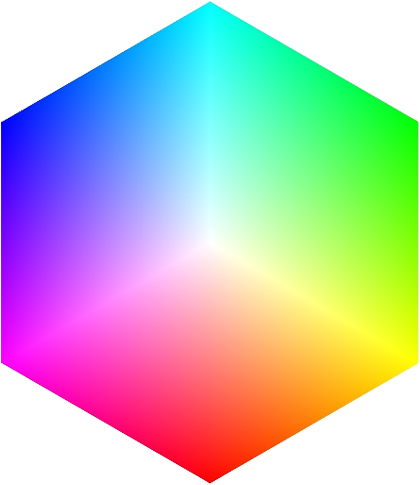60 degrees
90 degrees
108 degrees
120 degrees

Four
Five
Six
Seven

What shape is a 50 cent coin?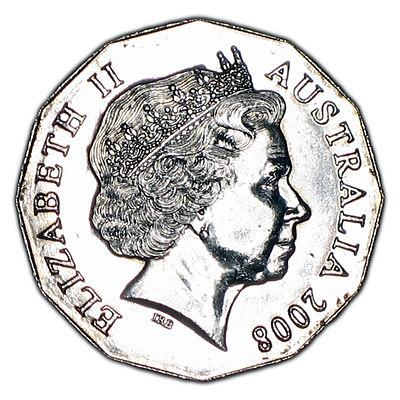Hexagon
Octagon
Decagon
Dodecagon

Which shape has 8 corners and sides?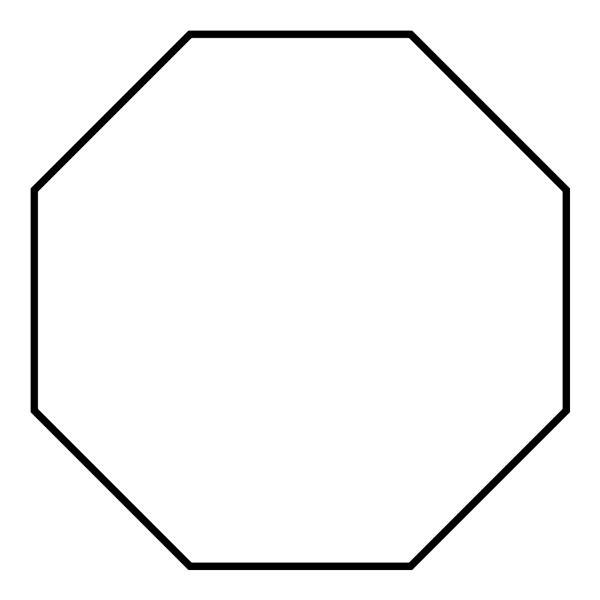Hexagon
Octagon
Decagon
Octakaidecagon

Which shape has four sides of equal length and four right angles?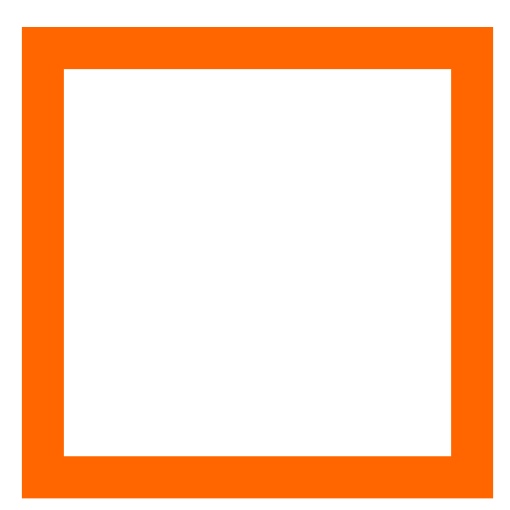Triangle
Square
Rectangle
Pentagon

True
False
Neither

A "trigon" is also known as a...

Triangle
Square
Shape with 13 sides
Shape with 30 sides

1,000
10,000
100,000
1,000,000

What type of angle is this?Right Angle
Obtuse Angle
Acute Angle
Reflex Angle

6
8
10
12